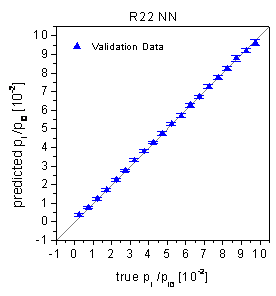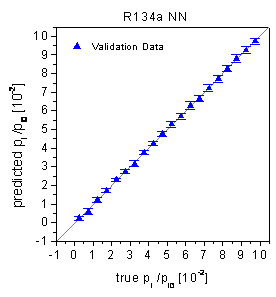Home News About Me Ph. D. Thesis Abstract Table of Contents 1. Introduction 2. Theory  Fundamentals of the Multivariate Data Analysis 3. Theory  Quantification of the Refrigerants R22 and R134a: Part I 4. Experiments, Setups and Data Sets 5. Results  Kinetic Measurements 6. Results  Multivariate Calibrations 6.1. PLS Calibration 6.2. Box-Cox Transformation + PLS 6.3. INLR 6.4. QPLS 6.5. CART 6.6. Model Trees 6.7. MARS 6.8. Neural Networks 6.9. PCA-NN 6.10. Neural Networks and Pruning 6.11. Conclusions 7. Results  Genetic Algorithm Framework 8. Results  Growing Neural Network Framework 9. Results  All Data Sets 10. Results  Various Aspects of the Frameworks and Measurements 11. Summary and Outlook 12. References 13. Acknowledgements Publications Research Tutorials Downloads and Links Contact Search Site Map Print this Page## 6.8.   Neural Networks

In this section, uniform fully connected feedforward neural networks are used for the calibration of the refrigerant data set with the calibration data set used for the training of the neural networks and the validation data set predicted by the trained networks. Separate networks were used for both analytes. For the training, the SCG algorithm with early stopping was used (see section 2.7.3). More details of the implementation of the networks and the learning algorithms are described in the sections 2.7.2 and 2.7.3. Different network topologies were systematically investi­gated by varying the number of hidden layers and the number of neurons in the hidden layer. The best topology for both analytes evaluated by the lowest crossvalidation error of the calibration data set are fully connected networks with 6 hidden neurons organized in 1 hidden layer. Twenty networks of this topology were trained using different random initial weights and the network with the lowest crossvalidation error was used for the prediction of the validation data. According to table 2, the validation data were predicted with relative RMSE of 2.18% (R22) respectively 3.26% (R134a). The predictions of the validation data (see figure 43) do not show any type of nonlinearity in conformity with the Wald-Wolfowitz Runs test and the Durbin-Watson statistics. Among the different calibration methods used for the refrigerant data set so far, the neural networks can model the nonlinear relationships between the concentrations of the analytes and the time-resolved sensor signals best resulting in the lowest errors of prediction of the validation data. Yet, the calibration data were predicted with significantly lower relative RMSE of 1.47% (R22) respectively 2.62% (R134a) indicating an overfitting. According to equation (13), the amount of 247 adjustable parameters of this network topology seems to be quite high for the calibration problem with many redundant or unused links resulting in overoptimistic low calibration errors but a decreased generalization ability. Thus, different methods like a variable selection and an optimization of the network topology are used in the next sections expecting that the reduction of the number of parameters further improves the generalization ability.figure 43:  Predictions of the validation data by fully connected neural networks.

 Page 97 © Frank Dieterle, 03.03.2019